Main Restorations Software Audio/Jukebox/MP3 Everything Else Buy/Sell/Trade Project Announcements Monitor/Video GroovyMAME Merit/JVL Touchscreen Meet Up Retail Vendors Driving & Racing Woodworking Software Support Forums Consoles Project Arcade Reviews Automated Projects Artwork Frontend Support Forums Pinball Forum Discussion Old Boards Raspberry Pi & Dev Board controls.dat Linux Miscellaneous Arcade Wiki Discussion Old Archives Lightguns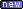Arcade1Up--- Bug Reports --- Site News Unread posts | New Replies | Recent posts | Rules | Chatroom | Wiki | File Repository | RSS | Submit news

###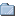Author Topic: Community (as in shared for free) Artwork Repository?  (Read 1455 times)

0 Members and 1 Guest are viewing this topic.

#### killallhumans

• Full Member
•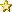•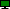Offline
•• Posts: 37
• Last login:August 14, 2019, 04:52:47 am
• What does this red button do?« on: January 31, 2019, 10:20:59 am »
What shall, we use, to fill, the empty, - spaces?

Can we have one place to share artwork?
Custom creations:
* Backdrops, bezels, menus, and elements. (pref *.zip file of 32-bit png layers)
* FrontEnd themes.
* Marquee, tabletop, cp, and side art.

I won't sell anything i've spent time drawing,
but i would let others freely use* them.

*Use: To copy, modify, or change, without any fee or credit required.

Sample of moving on-screen joystick in tall (768x1366) PacMan.lay
This bezel trick works for shifters, status lights, and other MAME outputs.
AutoIt 3 fixes everything.
control mapping, screen rotation...
Compile scripts to EXEs and drop them

#### killallhumans

• Full Member
••Offline
•• Posts: 37
• Last login:August 14, 2019, 04:52:47 am
• What does this red button do?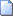« Reply #1 on: January 31, 2019, 10:31:24 am »
<!-- pacman.lay -->

<mamelayout version="2">
<element name="backdrop">
<image file="main.png" />
</element>
<element name="blue_ctr">
<image file="blue_ctr.png">
<bounds left="0" right="120" top="0" bottom="120" />
</image>
</element>
<element name="js1_0x01" defstate="0">
<image file="blue_up.png" state="0">
<bounds left="0" right="120" top="0" bottom="120" />
<color alpha="0.0" />
</image>
<image file="blue_up.png" state="1">
<bounds left="0" right="120" top="0" bottom="120" />
<color alpha="1.0" />
</image>
</element>
<element name="js1_0x02" defstate="0">
<image file="blue_left.png" state="0">
<bounds left="0" right="120" top="0" bottom="120" />
<color alpha="0.0" />
</image>
<image file="blue_left.png" state="1">
<bounds left="0" right="120" top="0" bottom="120" />
<color alpha="1.0" />
</image>
</element>
<element name="js1_0x04" defstate="0">
<image file="blue_right.png" state="0">
<bounds left="0" right="120" top="0" bottom="120" />
<color alpha="0.0" />
</image>
<image file="blue_right.png" state="1">
<bounds left="0" right="120" top="0" bottom="120" />
<color alpha="1.0" />
</image>
</element>
<element name="js1_0x08" defstate="0">
<image file="blue_down.png" state="0">
<bounds left="0" right="120" top="0" bottom="120" />
<color alpha="0.0" />
</image>
<image file="blue_down.png" state="1">
<bounds left="0" right="120" top="0" bottom="120" />
<color alpha="1.0" />
</image>
</element>

<element name="red_ctr">
<image file="red_ctr.png">
<bounds left="0" right="120" top="0" bottom="120" />
</image>
</element>
<element name="js0_0x01" defstate="0">
<image file="red_up.png" state="0">
<bounds left="0" right="120" top="0" bottom="120" />
<color alpha="0.0" />
</image>
<image file="red_up.png" state="1">
<bounds left="0" right="120" top="0" bottom="120" />
<color alpha="1.0" />
</image>
</element>
<element name="js0_0x02" defstate="0">
<image file="red_left.png" state="0">
<bounds left="0" right="120" top="0" bottom="120" />
<color alpha="0.0" />
</image>
<image file="red_left.png" state="1">
<bounds left="0" right="120" top="0" bottom="120" />
<color alpha="1.0" />
</image>
</element>
<element name="js0_0x04" defstate="0">
<image file="red_right.png" state="0">
<bounds left="0" right="120" top="0" bottom="120" />
<color alpha="0.0" />
</image>
<image file="red_right.png" state="1">
<bounds left="0" right="120" top="0" bottom="120" />
<color alpha="1.0" />
</image>
</element>
<element name="js0_0x08" defstate="0">
<image file="red_down.png" state="0">
<bounds left="0" right="120" top="0" bottom="120" />
<color alpha="0.0" />
</image>
<image file="red_down.png" state="1">
<bounds left="0" right="120" top="0" bottom="120" />
<color alpha="1.0" />
</image>
</element>

<view name="Generic_Artwork">
<screen index="0">
<bounds x="0" y="171" width="768" height="1024" />
</screen>
<bezel element="blue_ctr">
<bounds x="324" y="25" width="120" height="120" />
<orientation rotate="180" />
<color alpha="1.0" />
</bezel>
<bounds x="324" y="25" width="120" height="120" />
<orientation rotate="180" />
<color alpha="1.0" />
</bezel>
<bounds x="324" y="25" width="120" height="120" />
<orientation rotate="180" />
<color alpha="1.0" />
</bezel>
<bounds x="324" y="25" width="120" height="120" />
<orientation rotate="180" />
<color alpha="1.0" />
</bezel>
<bounds x="324" y="25" width="120" height="120" />
<orientation rotate="180" />
<color alpha="1.0" />
</bezel>
<bezel element="red_ctr">
<bounds x="324" y="1221" width="120" height="120" />
<color alpha="1.0" />
</bezel>
<bounds x="324" y="1221" width="120" height="120" />
<color alpha="1.0" />
</bezel>
<bounds x="324" y="1221" width="120" height="120" />
<color alpha="1.0" />
</bezel>
<bounds x="324" y="1221" width="120" height="120" />
<color alpha="1.0" />
</bezel>
<bounds x="324" y="1221" width="120" height="120" />
<color alpha="1.0" />
</bezel>
<backdrop element="backdrop">
<bounds x="0" y="0" width="768" height="1366" />
</backdrop>
</view>
</mamelayout>
AutoIt 3 fixes everything.
control mapping, screen rotation...
Compile scripts to EXEs and drop them

#### killallhumans

• Full Member
••Offline
•• Posts: 37
• Last login:August 14, 2019, 04:52:47 am
• What does this red button do?« Reply #2 on: February 23, 2019, 02:14:09 pm »
[bump]
Welcome,

Thank you, 200+ people, for reading.
Any thoughts?
Would board moderator like to comment?
AutoIt 3 fixes everything.